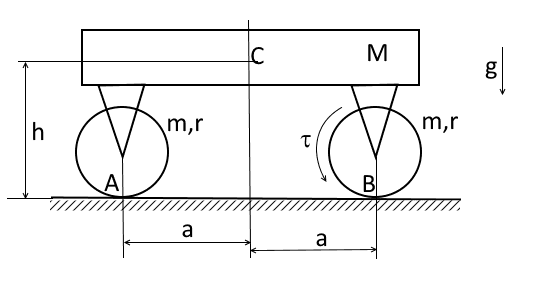# Force on wheels in an accelerating vehicle

• ein_stein

#### ein_stein

Assume that a motorcycle of mass m has two wheels that are equidistant from its centre i.e the force on each wheel is m*g/2.

If the motorcycle accelerates forward, will the two forces on each wheel (measured instantaneously) remain the same? If not, how can one mathematically describe the change in forces measured on each wheel and will these forces oscillate before converging when the motorcycle reaches final velocity?

Now, if the weight of the motorcycle isn't uniformly distributed along it's length e.g force measured at one wheel is say m*g/3 and 2*m*g/3 at the other, how different would the vehicle dynamics (oscillations etc) be? Can one somehow infer the fact that the weight isn't uniformly distributed without actually measuring the forces at the wheels?

I know this question is very vague but any ideas are very welcome.

I know this question is very vague but any ideas are very welcome.
Torque from driving the rear wheel takes weight off the front wheel, with high torque drivetrain the front wheel can come off the ground!

•billy_joule and HallsofIvy
In addition, even a front-wheel-drive car will experience less weight on the front wheels, and more weight on the rear when it accelerates.

If the motorcycle accelerates forward, will the two forces on each wheel (measured instantaneously) remain the same?

No.

If not, how can one mathematically describe the change in forces measured on each wheel and will these forces oscillate before converging when the motorcycle reaches final velocity?

The motorcycle accelerates due to the increased friction force acting on the rear wheel at ground level. This is below the centre of mass so you can think of this as a torque that tends to rotate the motorcycle in a direction that lifts the front wheel. Weight is transferred to the rear wheel until the torque sums to zero. If the torque did not sum to zero the bike would keep rotating until the front wheel is off the ground and beyond.

The force on each wheel won't oscillate. It will be roughly proportional to the acceleration. A motorbike cannot accelerate at a constant rate indefinitely (because it has limited power) so as it approaches max speed the acceleration reduces towards zero. The force on each wheel won't quite return to mg/2 because drag/air resistance also causes a torque that has to be balanced.

Quite tutorial task by the way. If one states it properly.Let us model the vehicle by means of a platform of mass M with center of mass at a point C and two wheels. Each wheel is a disk of radius r and of mass m. Other sizes are presented at the picture. The wheels can rotate without slipping at their contact points A, B. The right wheel experiences the torque ##\tau=const## from the motor stated on the vehicle .
Find the reaction forces ##\boldsymbol R_A,\boldsymbol R_B## between the ground and the wheels.